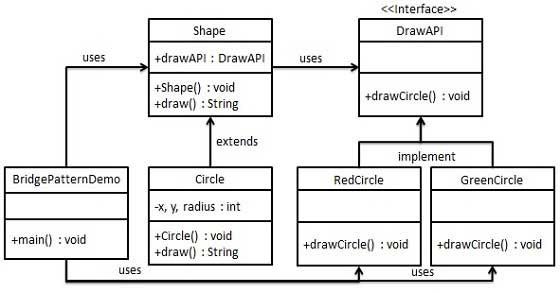Design Patterns - Bridge Pattern

Bridge is used when we need to decouple an abstraction from its implementation so that the two can vary independently. This type of design pattern comes under structural pattern as this pattern decouples implementation class and abstract class by providing a bridge structure between them.
This pattern involves an interface which acts as a bridge which makes the functionality of concrete classes independent from interface implementer classes. Both types of classes can be altered structurally without affecting each other.
We are demonstrating use of Bridge pattern via following example in which a circle can be drawn in different colors using same abstract class method but different bridge implementer classes.

Implementation

We have a DrawAPI interface which is acting as a bridge implementer and concrete classes RedCircleGreenCircle implementing the DrawAPI interface. Shape is an abstract class and will use object of DrawAPIBridgePatternDemo, our demo class will use Shape class to draw different colored circle.Step 1

Create bridge implementer interface.
DrawAPI.java
```public interface DrawAPI {
public void drawCircle(int radius, int x, int y);
}```

Step 2

Create concrete bridge implementer classes implementing the DrawAPIinterface.
RedCircle.java
```public class RedCircle implements DrawAPI {
@Override
public void drawCircle(int radius, int x, int y) {
System.out.println("Drawing Circle[ color: red, radius: " + radius + ", x: " + x + ", " + y + "]");
}
}```
GreenCircle.java
```public class GreenCircle implements DrawAPI {
@Override
public void drawCircle(int radius, int x, int y) {
System.out.println("Drawing Circle[ color: green, radius: " + radius + ", x: " + x + ", " + y + "]");
}
}```

Step 3

Create an abstract class Shape using the DrawAPI interface.
Shape.java
```public abstract class Shape {
protected DrawAPI drawAPI;

protected Shape(DrawAPI drawAPI){
this.drawAPI = drawAPI;
}
public abstract void draw();
}```

Step 4

Create concrete class implementing the Shape interface.
Circle.java
```public class Circle extends Shape {

public Circle(int x, int y, int radius, DrawAPI drawAPI) {
super(drawAPI);
this.x = x;
this.y = y;
}

public void draw() {
}
}```

Step 5

Use the Shape and DrawAPI classes to draw different colored circles.
BridgePatternDemo.java
```public class BridgePatternDemo {
public static void main(String[] args) {
Shape redCircle = new Circle(100,100, 10, new RedCircle());
Shape greenCircle = new Circle(100,100, 10, new GreenCircle());

redCircle.draw();
greenCircle.draw();
}
}```

Step 6

Verify the output.
```Drawing Circle[ color: red, radius: 10, x: 100, 100]
Drawing Circle[  color: green, radius: 10, x: 100, 100]```